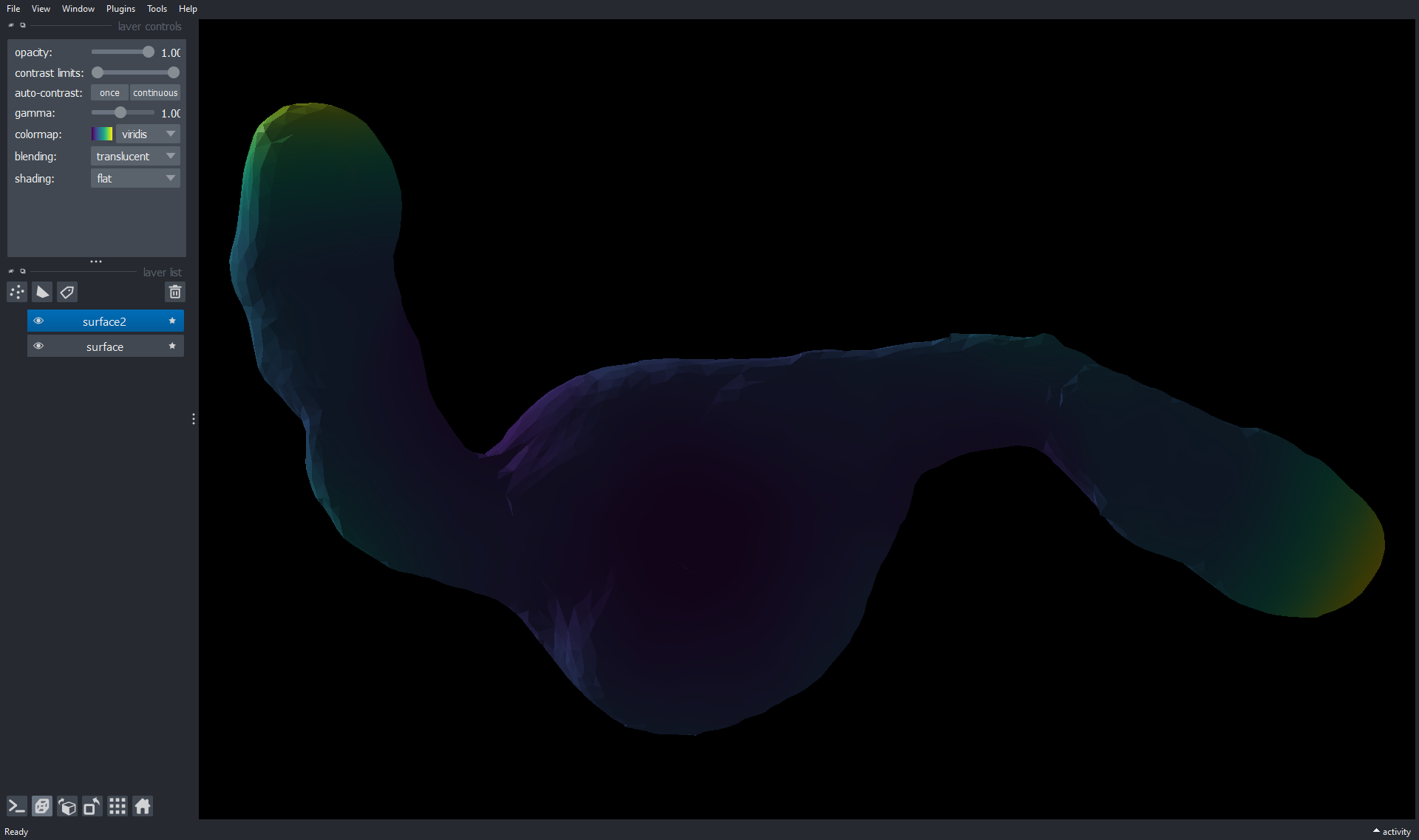# Visualizing surfaces#

In this notebook we create a surface mesh from a 3D dataset of a Gastruloid. The used example data is a derived from of AV Luque and JV Veenvliet (2023) licensed CC-BY. See the creating_surfaces for how to create the surface from raw imaging data.

We visualize the surface using napari-process-points-and-surfaces, vedo and napari.

import napari_process_points_and_surfaces as nppas

import vedo
import napari

surface = nppas.gastruloid()

The nppas gastruloid example is derived from AV Luque and JV Veenvliet (2023) which is licensed CC-BY (https://creativecommons.org/licenses/by/4.0/legalcode) and can be downloaded from here: https://zenodo.org/record/7603081


The resulting object is visualized in Jupyter notebooks like this:

surface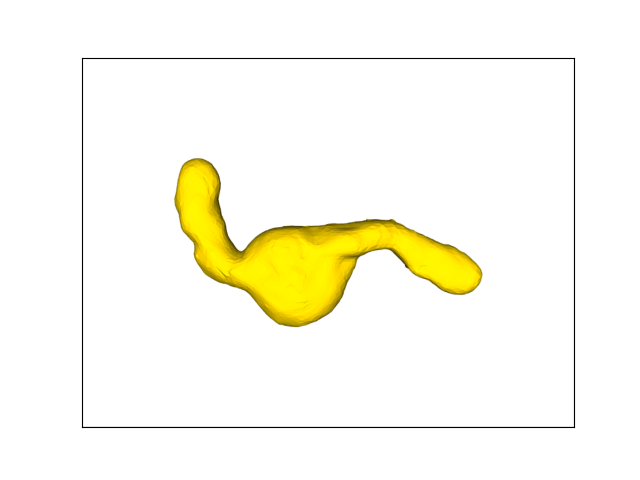nppas.SurfaceTuple
 origin (z/y/x) [0. 0. 0.] center of mass(z/y/x) 57.561,308.175,440.144 scale(z/y/x) 1.000,1.000,1.000 bounds (z/y/x) 13.983...112.865110.951...460.844168.949...807.707 average size 171.204 number of vertices 3324 number of faces 6643
surface2 = nppas.add_quality(surface, nppas.Quality.SPHERE_FITTED_CURVATURE_HECTA_VOXEL)
surface2.azimuth = -90
surface2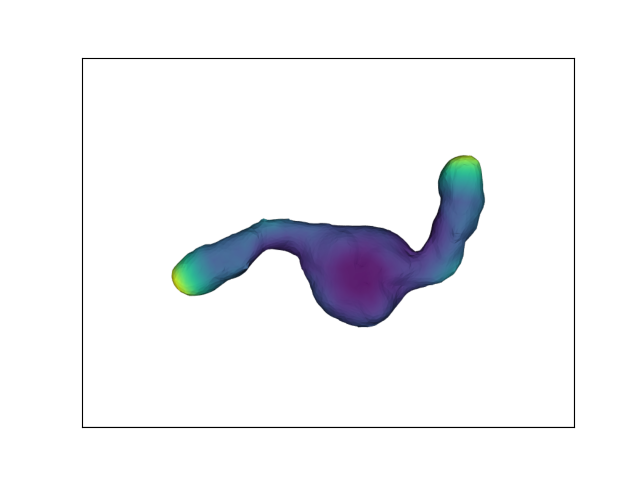nppas.SurfaceTuple
 origin (z/y/x) [0. 0. 0.] center of mass(z/y/x) 57.561,308.175,440.144 scale(z/y/x) 1.000,1.000,1.000 bounds (z/y/x) 13.983...112.865110.951...460.844168.949...807.707 average size 171.204 number of vertices 3324 number of faces 6643 min 0.00015164456647196744 max 0.0005448766287854695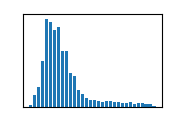## Visualizing surfaces using napari-process-points-and-surfaces nazimuths.show()#

You can tune the visualization using nppas.show().

nppas.show(surface, zoom=1.5, azimuth=90, elevation=45)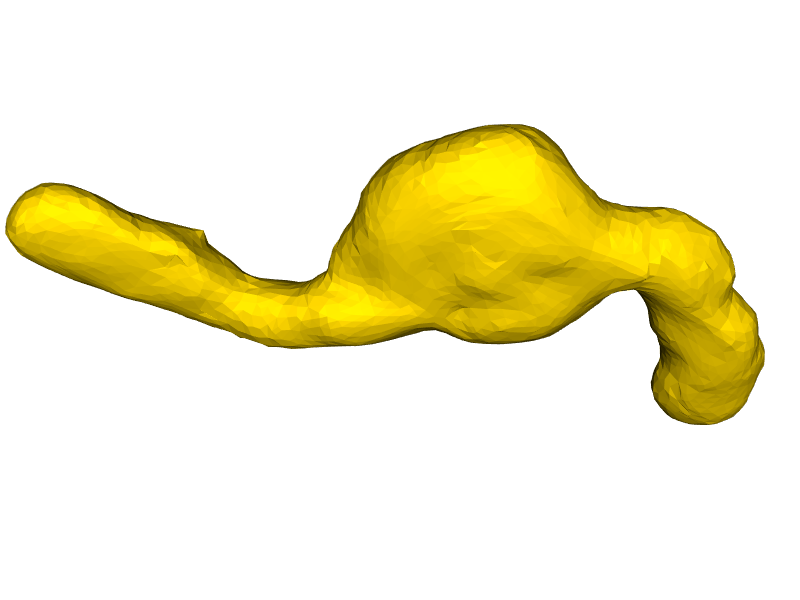## Visualizing surfaces using vedo#

… using vedo Plotter.

from vedo import Plotter

plt = Plotter()
mesh = nppas.to_vedo_mesh(surface)

plt.show(mesh, zoom=1, azimuth=45)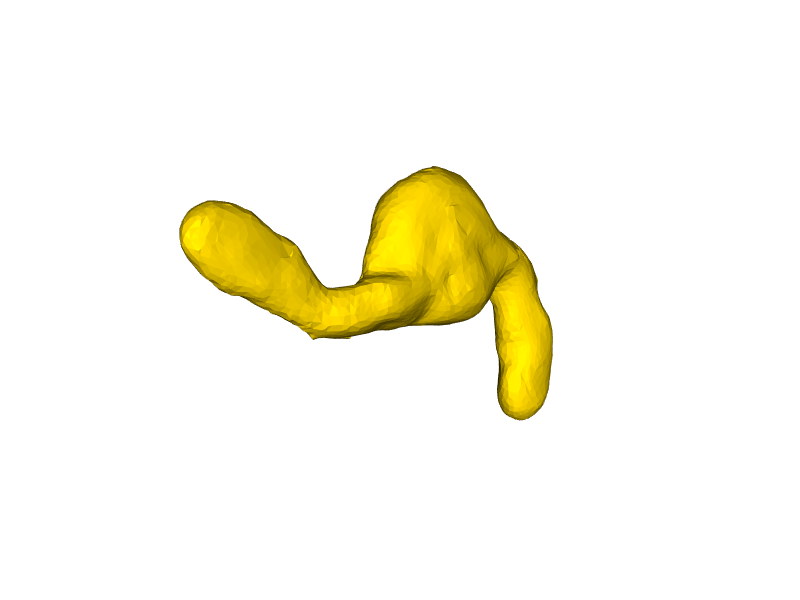## Visualizing surfaces using napari#

import napari
viewer = napari.Viewer(ndisplay=3)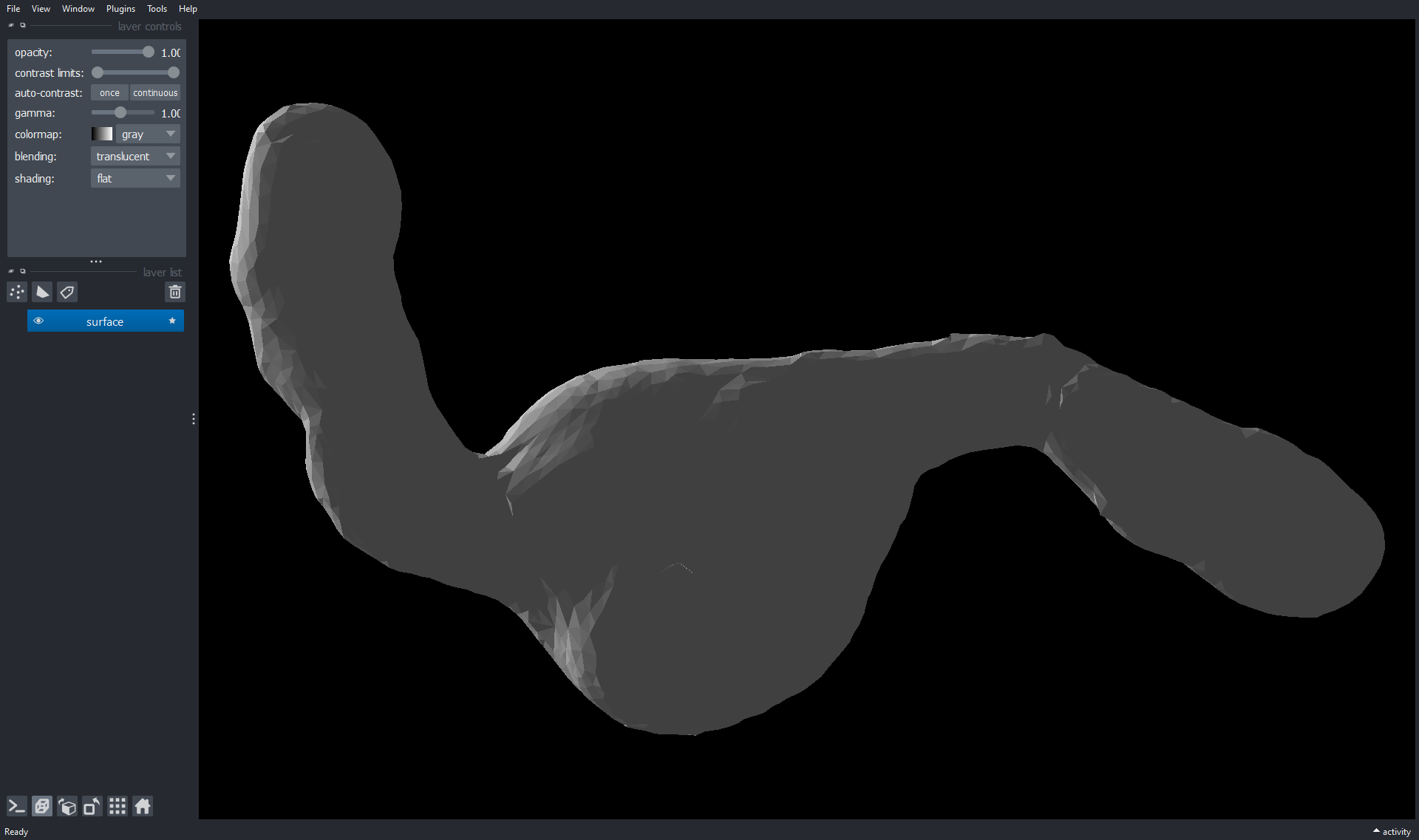viewer.add_surface(surface2, colormap=surface2.cmap)